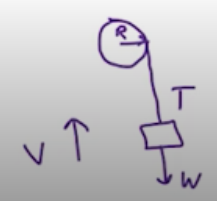Need Help?

Subscribe to Physics 1

###### \${selected_topic_name}
• Notes

$\begin{array}{l}{\text { A } 1.50-\mathrm{kg} \text { grinding wheel is in the form of a solid cylinder }} \\ {\text { of radius } 0.100 \mathrm{m} \text { . (a) What constant torque will bring it from rest }} \\ {\text { to an angular speed of } 1200 \mathrm{rev} / \min \text { in } 2.5 \mathrm{s} \text { ? (b) Through what }} \\ {\text { angle has it turned during that time? (c) Use Eq. }(10.21) \text { to calcu- }} \\ {\text { late the work done by the torque. (d) What is the grinding wheel's }} \\ {\text { kinetic energy when it is rotating at } 1200 \mathrm{rev} / \mathrm{min} \text { ? Compare your }} \\ {\text { answer to the result in part (c). }}\end{array}$

$\sum \tau_{z}=I \alpha_{z} \quad , \quad 1 rev=2 \pi \ rad \quad , \quad \pi \ rad/s=30 \ rev/min$

$\tau_z=I \alpha_{z}=I \frac{\omega_{z}-\omega_{0 z}}{t}$

$=\frac{\left(\frac{1}{2}(1.5)(0.1)^{2}\right)(1200 \mathrm{rev/min})\left(\frac{\pi}{30}\right)}{2.5}=0.377 \mathrm{N} \cdot \mathrm{m}$

$\omega_{\text {av }} \Delta t=\frac{600(2.5)}{60}=25 \mathrm{rev}=157 \mathrm{rad}$

$w=\tau \Delta \theta=0.377(157)=59.2J$

$k=\frac{1}{2} I \omega^{2}=\frac{1}{2} (\frac{1}{2}(1.5)(0.1)^{2})\left[1200\left(\frac{\pi}{30}\right)\right]=59.2 J$

$\begin{array}{l}{\text { (a) Compute the torque developed by an industrial motor }} \\ {\text { whose output is } 150 \mathrm{kW} \text { at an angular speed of } 4000 \text { rev/min. }} \\ {\text { (b) A drum with negligible mass, } 0.400 \mathrm{m} \text { in diameter, is attached }} \\ {\text { to the motor shaft, and the power output of the motor is used to }} \\ {\text { raise a weight hanging from a rope wrapped around the drum. How }} \\ {\text { heavy a weight can the motor lift at constant speed? (c) At what }} \\ {\text { constant speed will the weight rise? }}\end{array}$

$P=\tau_{z} w_{z}$

$\omega_{z}=4000 \text { rev/min}\left(\frac{2 \pi}{60}\right)=418.9 \mathrm{rad} / \mathrm{s}$

$\tau_{z}=\frac{p}{\omega_{z}}=\frac{1.5 * 10^{5} w}{418.9}=358 \mathrm{N} \cdot \mathrm{m}$

$\sum \vec{F}=m \vec{a}$$T-w =0 \longrightarrow T=w$

$\tau_{z}=T{R} \rightarrow T=\frac{\tau_{z}}{R}=\frac{358}{0.2}=1790 \mathrm{N}$

$w=1790 \mathrm{N}$        can be lifted

$V=R \omega=0.2(418.9)=83.8 \mathrm{m/s}$

$P=T. V=1790 * 83.8=150 \mathrm{kw}$